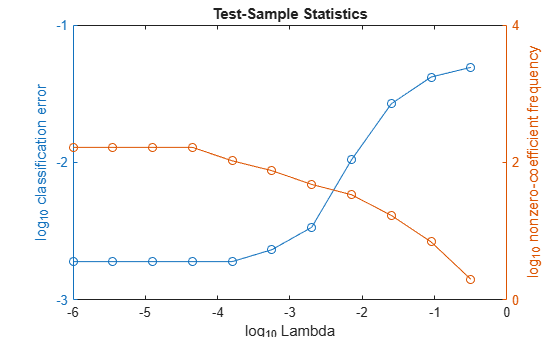Main Content

# selectModels

Class: ClassificationLinear

Choose subset of regularized, binary linear classification models

## Syntax

``SubMdl = selectModels(Mdl,idx)``

## Description

example

````SubMdl = selectModels(Mdl,idx)` returns a subset of trained, binary linear classification models from a set of binary linear classification models (`Mdl`) trained using various regularization strengths. The indices (`idx`) correspond to the regularization strengths in `Mdl.Lambda`, and specify which models to return.```

## Input Arguments

expand all

Binary linear classification models trained using various regularization strengths, specified as a `ClassificationLinear` model object. You can create a `ClassificationLinear` model object using `fitclinear`.

Although `Mdl` is one model object, if `numel(Mdl.Lambda)` = L ≥ 2, then you can think of `Mdl` as L trained models.

Indices corresponding to regularization strengths, specified as a numeric vector of positive integers. Values of `idx` must be in the interval [1,L], where L = `numel(Mdl.Lambda)`.

Data Types: `double` | `single`

## Output Arguments

expand all

Subset of binary linear classification models trained using various regularization strengths, returned as a `ClassificationLinear` model object.

## Examples

expand all

To determine a good lasso-penalty strength for a linear classification model that uses a logistic regression learner, compare test-sample classification error rates.

Load the NLP data set. Preprocess the data as in Specify Custom Classification Loss.

```load nlpdata Ystats = Y == 'stats'; X = X'; rng(10); % For reproducibility Partition = cvpartition(Ystats,'Holdout',0.30); testIdx = test(Partition); XTest = X(:,testIdx); YTest = Ystats(testIdx);```

Create a set of 11 logarithmically-spaced regularization strengths from $1{0}^{-6}$ through $1{0}^{-0.5}$.

`Lambda = logspace(-6,-0.5,11);`

Train binary, linear classification models that use each of the regularization strengths. Optimize the objective function using SpaRSA. Lower the tolerance on the gradient of the objective function to `1e-8`.

```CVMdl = fitclinear(X,Ystats,'ObservationsIn','columns',... 'CVPartition',Partition,'Learner','logistic','Solver','sparsa',... 'Regularization','lasso','Lambda',Lambda,'GradientTolerance',1e-8)```
```CVMdl = ClassificationPartitionedLinear CrossValidatedModel: 'Linear' ResponseName: 'Y' NumObservations: 31572 KFold: 1 Partition: [1x1 cvpartition] ClassNames: [0 1] ScoreTransform: 'none' Properties, Methods ```

Extract the trained linear classification model.

`Mdl = CVMdl.Trained{1}`
```Mdl = ClassificationLinear ResponseName: 'Y' ClassNames: [0 1] ScoreTransform: 'logit' Beta: [34023x11 double] Bias: [1x11 double] Lambda: [1x11 double] Learner: 'logistic' Properties, Methods ```

`Mdl` is a `ClassificationLinear` model object. Because `Lambda` is a sequence of regularization strengths, you can think of `Mdl` as 11 models, one for each regularization strength in `Lambda`.

Estimate the test-sample classification error.

`ce = loss(Mdl,X(:,testIdx),Ystats(testIdx),'ObservationsIn','columns');`

Because there are 11 regularization strengths, `ce` is a 1-by-11 vector of classification error rates.

Higher values of `Lambda` lead to predictor variable sparsity, which is a good quality of a classifier. For each regularization strength, train a linear classification model using the entire data set and the same options as when you cross-validated the models. Determine the number of nonzero coefficients per model.

```Mdl = fitclinear(X,Ystats,'ObservationsIn','columns',... 'Learner','logistic','Solver','sparsa','Regularization','lasso',... 'Lambda',Lambda,'GradientTolerance',1e-8); numNZCoeff = sum(Mdl.Beta~=0);```

In the same figure, plot the test-sample error rates and frequency of nonzero coefficients for each regularization strength. Plot all variables on the log scale.

```figure; [h,hL1,hL2] = plotyy(log10(Lambda),log10(ce),... log10(Lambda),log10(numNZCoeff + 1)); hL1.Marker = 'o'; hL2.Marker = 'o'; ylabel(h(1),'log_{10} classification error') ylabel(h(2),'log_{10} nonzero-coefficient frequency') xlabel('log_{10} Lambda') title('Test-Sample Statistics') hold off```Choose the index of the regularization strength that balances predictor variable sparsity and low classification error. In this case, a value between $1{0}^{-4}$ to $1{0}^{-1}$ should suffice.

`idxFinal = 7;`

Select the model from `Mdl` with the chosen regularization strength.

`MdlFinal = selectModels(Mdl,idxFinal);`

`MdlFinal` is a `ClassificationLinear` model containing one regularization strength. To estimate labels for new observations, pass `MdlFinal` and the new data to `predict`.

## Tips

One way to build several predictive, binary linear classification models is:

1. Hold out a portion of the data for testing.

2. Train a binary, linear classification model using `fitclinear`. Specify a grid of regularization strengths using the `'``Lambda``'` name-value pair argument and supply the training data. `fitclinear` returns one `ClassificationLinear` model object, but it contains a model for each regularization strength.

3. To determine the quality of each regularized model, pass the returned model object and the held-out data to, for example, `loss`.

4. Identify the indices (`idx`) of a satisfactory subset of regularized models, and then pass the returned model and the indices to `selectModels`. `selectModels` returns one `ClassificationLinear` model object, but it contains `numel(idx)` regularized models.

5. To predict class labels for new data, pass the data and the subset of regularized models to `predict`.

## See Also

Introduced in R2016a

Download ebook